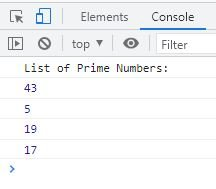# JavaScript filter() | Filter an Array Based on Condition

The JavaScript filter() method is used when we need to check every element in an array to find if it meets the specified condition and create a new array with only those elements that meets the specified condition. For example:

HTML with JavaScript Code
```<!DOCTYPE html>
<html>
<body>

<p id="xyz"></p>

<script>
const mynumbers = [12, 32, 43, 5, 65, 46];

document.getElementById("xyz").innerHTML = mynumbers.filter(even);

function even(x)
{
return x%2==0;
}
</script>

</body>
</html>```
Output

As you can see from above example, using the filter() method, I've filtered the array mynumbers with even numbers.

Note - The original array does not get effected by the filter() method.

## JavaScript filter() Syntax

The syntax of filter() method in JavaScript is:

`array.filter(functionName(currentElementValue, currentElementIndex, currentElementArray), thisValue)`

The array, functionName, and currentElementValue are required. All others are optional.

Note - The array refers to an array that is going to filter, using the function functionName.

Note - The functionName refers to a function to execute for every elements of array.

Note - The currentElementValue basically refers to a variable that will be used to pass as an argument to the function that of course indicates to the current value/element of the specified array.

Note - The currentElementIndex refers to the index of the current element

Note - The currentElementArray refers to the array of the current element.

Note - The thisValue refers to a value passed to the specified function functionName as its this value. The default value is undefined

## JavaScript filter() Array Example

Let me create an example of filter() method that uses a function with three parameters:

HTML with JavaScript Code
```<!DOCTYPE html>
<html>
<body>

<p>Odd Numbers: <span id="abc"></span></p>

<script>
const a = [12, 32, 43, 5, 65, 46, 19, 17, 48];

const o = a.filter(odd);

function odd(num, indx, arr)
{
if(indx==0 || indx==(arr.length-1))
return true;

return num%2!=0;
}

document.getElementById("abc").innerHTML = o;

</script>

</body>
</html>```
Output

Odd Numbers:

In above example, the first and last numbers are even numbers, that are 12 and 48, but I've filtered the array a to filter all elements except the element at first and last index.

Here is another example of filter() function that filters an array based on prime numbers.

HTML with JavaScript Code
```<!DOCTYPE html>
<html>
<body>

<script>
const nums = [12, 32, 43, 5, 65, 46, 19, 17];

function prime(num)
{
let count=0;
for(let i=2; i<num; i++)
{
if(num%i == 0)
{
count++;
break;
}
}
if(count==0)
return true;
else
return false;
}

console.log("No prime numbers found in the array");
else
{
console.log("List of Prime Numbers:");
}
</script>

</body>
</html>```

The snapshot given below shows the sample output produced by above example:JavaScript Online Test

« Previous Tutorial Next Tutorial »